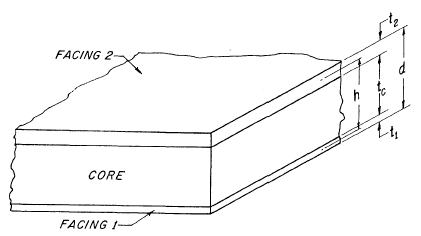Cored Laminate Composite Stiffness Equations & Calculator

Cored Laminate Composite Stiffness Equations and Calculator

In the development of formulas for deflection, stresses , and buckling of sandwich components, mathematical expressions for bending, extensional, and shear stiffness often appear as do parameters involving these stiffnesses. It is convenient to present the fundamental stiffness formulas at the outset. Here also are discussed the effects of facing and core stiffness on sandwich bending stiffness so that the degree of approximation implied by simplified formulas neglecting facing and core stiffness is known.

For more detail information see: Structural Sandwich Composites Design ManualA structural sandwich under forces normal to its facings has a bending stiffness, per unit width, given by the equation:

Where:

Sandwich Bending Stiffness:

The extensional stiffness of a sandwich, stretched or compressed by force in its plane, is given by the formula.

D = Bending Stiffness, lb-in2E'n is the effective modulus of elasticity of facing, psi

E'c is effective core elastic modulus in the appropriate L or W direction, psi

Vn= Poissons Ratio

VnC= Poissons Ratio, Core1 and 2 are subscripts denoting facing 1 and 2

Sandwich Extensional Stiffness:This Calculator requires a Premium Membership to access

References: MIL-HDBK-23Membership Register | LoginHomeEngineering Book StoreEngineering ForumExcel App. DownloadsOnline Books & ManualsEngineering NewsEngineering VideosEngineering CalculatorsEngineering ToolboxGD&T Training Geometric Dimensioning TolerancingDFM DFA TrainingTraining Online EngineeringAdvertising CenterCopyright Notice